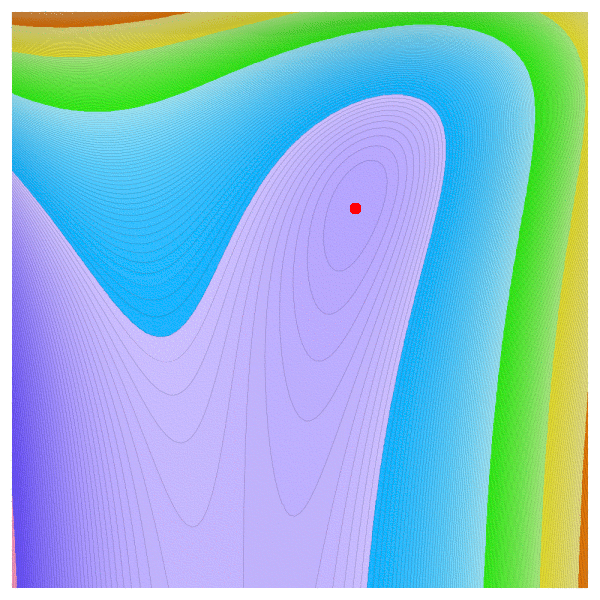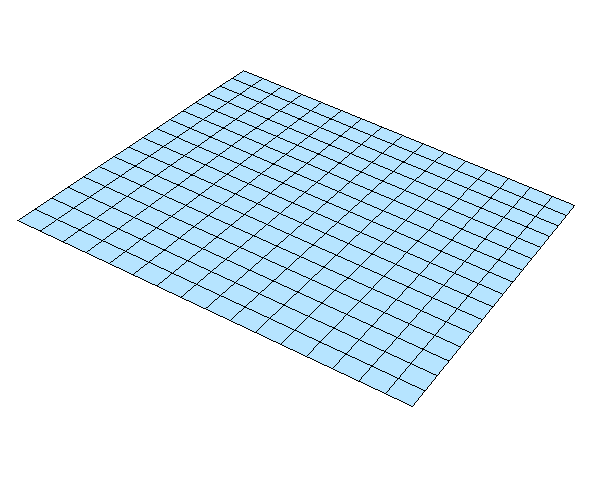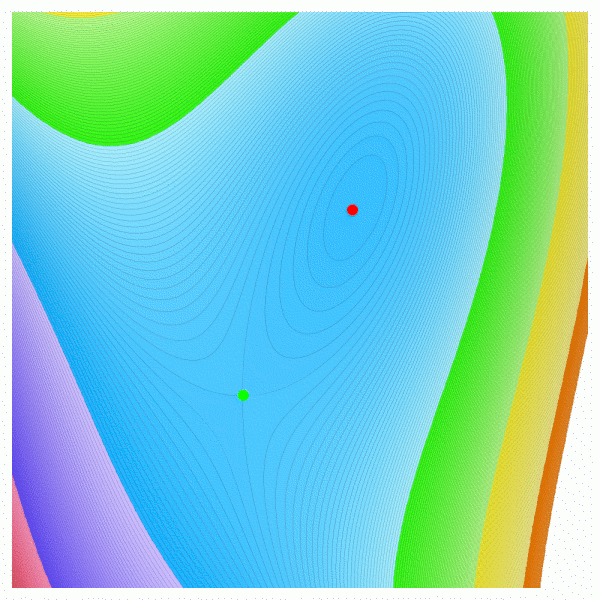Math 21a, Fall 2007
Exhibits page Math 21a 07, Multivariable Calculus
Global and local extremaIn single variable calculus it is known that if a smooth function f(x) is unbounded below and above and has a local maximum at a point c, then it also must have a local minimum. Is there a function f(x,y) is unbounded below and above and has exactly one local minimum? One would think that there is an additional saddle point (mountain pass), one has to climb over to get from the local minimum to the region lying lower than this local minimum. But the answer is Yes: here is an example given by Chen-Yu Chi: ```f(x,y)=x3+ e3 y - 3 x ey ``` The function has exactly one critical point and as one can see with y=0 is unbounded above and below. Here is a challenge: can one find a polynomial f(x,y) for which there exists exactly one local minimum but where the function is unbounded above and below?This problem came up while grading one of the exam problems for the second midterm. Many students argued in problem 8 that if there exists only one critical point which is a local minimum, then it is automatically a global minimum. This is not correct.We had a little discussion and I (Oliver) thought that one could get an example of a single local non-global extremum by taking a plane like z=x+y and carve a little "dip" into it to get a local extremum. The animation illustrates this with f(x,y) = x+y- c exp(-x2-y2) for various values of c. Other team members objected that this would not produce a counter example and indeed we always see a second saddle point. The idea does not work, In order to get an example as above, the saddle has to be moved to infinity. And Chen-Yu's example does this.How did Chen-Yu construct the example? He started with the function ```g(x,y) = x^3+ y^3 - 3 x y ``` which has two critical points, a minimum (1,1) and a saddle point at (0,0). He then looked at the function f(x,y) = g(x,ey. The picture to the left shows the level curves of the function g(x,y). Replacing y with ey has the effect that the saddle point of g(x,y) is "pushed" to infinity.